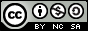- 目录 -
LeetCode 560 和为K的子数组(前缀和，思路)

输入:nums = [1,1,1], k = 2



1. 数组的长度为 [1, 20,000]。
2. 数组中元素的范围是 [-1000, 1000] ，且整数 k 的范围是 [-1e7, 1e7]。

## 代码

  1 2 3 4 5 6 7 8 9 10 11 12 13 14 15 16 17  class Solution { public: int subarraySum(vector &nums, int k) { unordered_map mp; mp = 1; long long sum = 0, ans = 0; for (int i = 1; i <= nums.size(); i++) { sum += nums[i - 1]; ans += mp[sum - k]; mp[sum]++; } return ans; } };